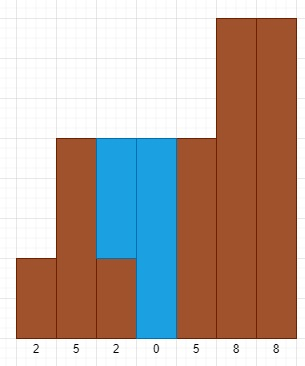# Program to find how many total amount of rain we can catch in Python

Suppose we have an array of n non-negative integers. These are representing a height where the width of each bar is 1, we have to compute how much water it is able to catch after raining. So the map will be like −Here we can see there are 8 blue boxes, so the output will be 8.

To solve this, we will follow these steps −

• Define a stack st, water := 0 and i := 0
• while i < size of height
• if is stack is empty or height[stack top] >= height[i], then push i into stack, increase i by 1
• otherwise
• x := stack top element, delete top from stack
• if stack is not empty, then
• temp := min of height[stack top element] and height[i]
• dest := i – stack top element – 1
• water := water + dist * (temp – height[x])
• return water

Let us see the following implementation to get better understanding −

## Example

Live Demo

class Solution(object):
def trap(self, height):
stack = []
water = 0
i=0
while i<len(height):
if len(stack) == 0 or height[stack[-1]]>=height[i]:
stack.append(i)
i+=1
else:
x = stack[-1]
stack.pop()
if len(stack) != 0:
temp = min(height[stack[-1]],height[i])
dist = i - stack[-1]-1
water += dist*(temp - height[x])
return water
ob = Solution()
print(ob.trap([2,5,2,0,5,8,8]))

## Input

[2,5,2,0,5,8,8]

## Output

8